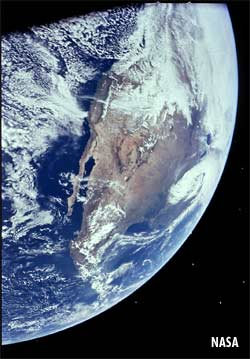QUESTION #419

# What is the volume of Earth?

The earth is approximately a sphere (actually it is sphere slightly flattened at the poles). Its volume can be calculated if you know its radius. Use the equation for the volume of a sphere which is V = 4/3x Radius3The mean radius of the earth is approximately 6.4 million meters (exact = 6.37 x 106 m). Its volume is then: